## Recommended Posts

Hello everyone,

I have been having a hard time with this equation...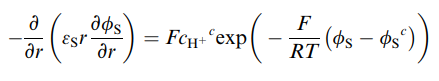I would really like to know if anybody has an idea on how to solve it...

All the suggestions are very much appreciated :)

##### Share on other sites

It would be a lot easier to understand and be sure of your notation if you provided a symbols key.

is FcH+c meant to be  (F) (cH+)c  ?

Have you plotted a direction or characteristic field for the equation?

##### Share on other sites

It loos like an ordinary (not partial) diff. eq. in r.  Which symbols represent functions of r?

##### Share on other sites

The following 6 symbols below are constants: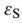...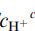...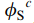... F... R... T...

And what I need is to solve the equation for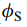, which varies with r.

Regarding your questions, I haven't plotted the characteristic field as I never heard about it...

Onlyis a function of r...

##### Share on other sites
4 hours ago, Miguel_s said:

The following 6 symbols below are constants:

And what I need is to solve the equation for, which varies with r.

Regarding your questions, I haven't plotted the characteristic field as I never heard about it...

Since you only have two variables I agree with mathematic that this is an ODE.

I would proceed as follows:

Replacing all your constants and collecting them together, you only need 3 constants.

I have started with four, A, B, C and D and finally added E = B/A

$- \frac{d}{{dr}}\left( {\varepsilon r\frac{{d\varphi }}{{dr}}} \right) = B{e^{ - \left( {C\varphi + D} \right)}}$

$- \varepsilon \frac{d}{{dr}}\left( {r\frac{{d\varphi }}{{dr}}} \right) = B{e^{ - \left( {C\varphi + D} \right)}}$

$A\frac{d}{{dr}}\left( {r\frac{{d\varphi }}{{dr}}} \right) = B{e^{ - \left( {C\varphi + D} \right)}}$

$A\left( {\frac{{d\varphi }}{{dr}} + r\frac{{{d^2}\varphi }}{{d{r^2}}}} \right) = B{e^{ - \left( {C\varphi + D} \right)}}$

$\left( {\frac{{d\varphi }}{{dr}} + r\frac{{{d^2}\varphi }}{{d{r^2}}}} \right) = E{e^{ - \left( {C\varphi + D} \right)}}$

##### Share on other sites
17 minutes ago, studiot said:

Since you only have two variables I agree with mathematic that this is an ODE.

I would proceed as follows:

Replacing all your constants and collecting them together, you only need 3 constants.

I have started with four, A, B, C and D and finally added E = B/A

ddr(εrdφdr)=Be(Cφ+D)

εddr(rdφdr)=Be(Cφ+D)

A(dφdr+rd2φdr2)=Be(Cφ+D)

(dφdr+rd2φdr2)=Ee(Cφ+D)

It definitely looks better. From that stage on is where I can't just go through... I have tried variable substitution before and nothing...

If you have any idea I would really appreciate

## Create an account

Register a new account